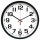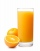# Fraction calculator

The calculator performs basic and advanced operations with fractions, expressions with fractions combined with integers, decimals, and mixed numbers. It also shows detailed step-by-step information about the fraction calculation procedure. Solve problems with two, three, or more fractions and numbers in one expression.

## Result:

### 41/2 - 11/3 = 19/6 = 3 1/6 ≅ 3.1666667

Spelled result in words is nineteen sixths (or three and one sixth).

### How do you solve fractions step by step?

1. Conversion a mixed number 4 1/2 to a improper fraction: 4 1/2 = 4 1/2 = 4 · 2 + 1/2 = 8 + 1/2 = 9/2

To find new numerator:
a) Multiply the whole number 4 by the denominator 2. Whole number 4 equally 4 * 2/2 = 8/2
b) Add the answer from previous step 8 to the numerator 1. New numerator is 8 + 1 = 9
c) Write a previous answer (new numerator 9) over the denominator 2.

Four and one half is nine halfs
2. Conversion a mixed number 1 1/3 to a improper fraction: 1 1/3 = 1 1/3 = 1 · 3 + 1/3 = 3 + 1/3 = 4/3

To find new numerator:
a) Multiply the whole number 1 by the denominator 3. Whole number 1 equally 1 * 3/3 = 3/3
b) Add the answer from previous step 3 to the numerator 1. New numerator is 3 + 1 = 4
c) Write a previous answer (new numerator 4) over the denominator 3.

One and one third is four thirds
3. Subtract: 9/2 - 4/3 = 9 · 3/2 · 3 - 4 · 2/3 · 2 = 27/6 - 8/6 = 27 - 8/6 = 19/6
For adding, subtracting, and comparing fractions, it is suitable to adjust both fractions to a common (equal, identical) denominator. The common denominator you can calculate as the least common multiple of both denominators - LCM(2, 3) = 6. In practice, it is enough to find the common denominator (not necessarily the lowest) by multiplying the denominators: 2 × 3 = 6. In the next intermediate step, the fraction result cannot be further simplified by canceling.
In words - nine halfs minus four thirds = nineteen sixths.

#### Rules for expressions with fractions:

Fractions - use the slash “/” between the numerator and denominator, i.e., for five-hundredths, enter 5/100. If you are using mixed numbers, be sure to leave a single space between the whole and fraction part.
The slash separates the numerator (number above a fraction line) and denominator (number below).

Mixed numerals (mixed fractions or mixed numbers) write as non-zero integer separated by one space and fraction i.e., 1 2/3 (having the same sign). An example of a negative mixed fraction: -5 1/2.
Because slash is both signs for fraction line and division, we recommended use colon (:) as the operator of division fractions i.e., 1/2 : 3.

Decimals (decimal numbers) enter with a decimal point . and they are automatically converted to fractions - i.e. 1.45.

The colon : and slash / is the symbol of division. Can be used to divide mixed numbers 1 2/3 : 4 3/8 or can be used for write complex fractions i.e. 1/2 : 1/3.
An asterisk * or × is the symbol for multiplication.
Plus + is addition, minus sign - is subtraction and ()[] is mathematical parentheses.
The exponentiation/power symbol is ^ - for example: (7/8-4/5)^2 = (7/8-4/5)2

#### Examples:

subtracting fractions: 2/3 - 1/2
multiplying fractions: 7/8 * 3/9
dividing Fractions: 1/2 : 3/4
exponentiation of fraction: 3/5^3
fractional exponents: 16 ^ 1/2
adding fractions and mixed numbers: 8/5 + 6 2/7
dividing integer and fraction: 5 ÷ 1/2
complex fractions: 5/8 : 2 2/3
decimal to fraction: 0.625
Fraction to Decimal: 1/4
Fraction to Percent: 1/8 %
comparing fractions: 1/4 2/3
multiplying a fraction by a whole number: 6 * 3/4
square root of a fraction: sqrt(1/16)
reducing or simplifying the fraction (simplification) - dividing the numerator and denominator of a fraction by the same non-zero number - equivalent fraction: 4/22
expression with brackets: 1/3 * (1/2 - 3 3/8)
compound fraction: 3/4 of 5/7
fractions multiple: 2/3 of 3/5
divide to find the quotient: 3/5 ÷ 2/3

The calculator follows well-known rules for order of operations. The most common mnemonics for remembering this order of operations are:
PEMDAS - Parentheses, Exponents, Multiplication, Division, Addition, Subtraction.
BEDMAS - Brackets, Exponents, Division, Multiplication, Addition, Subtraction
BODMAS - Brackets, Of or Order, Division, Multiplication, Addition, Subtraction.
GEMDAS - Grouping Symbols - brackets (){}, Exponents, Multiplication, Division, Addition, Subtraction.
Be careful, always do multiplication and division before addition and subtraction. Some operators (+ and -) and (* and /) has the same priority and then must evaluate from left to right.

## Fractions in word problems:

• AkpanAkpan spent 3/8 of his time in school during the week. What fraction of his time does he spend at home during the week?
• Mixed numbersRewrite mixed numbers, so the fractions have the same denominator: 5 1/5 - 2 2/3
• Magic bagEach time the prince crossed the bridge, the number of tolars in the magic bag doubled. But then the devil always conjured 300 tolars for him. When this happened for the third time, the prince had twice as much as he had in the beginning. How many tolars
• SavingsEva borrowed 1/3 of her savings to her brother, 1/2 of savings spent in the store and 7 euros left. How much did she save?
• Evaluate - lowest termsEvaluate: 16/25 - 11/25 (Express answer as a fraction reduced to lowest terms. )
• PatelPatel squeezed oranges so that his family could have fresh-squeezed juice for breakfast. He squeezed 4/17 cups from the first orange, 3/10 cups from the second orange, StartFraction 9 over 20 E
• Empty and fullAn empty can has a mass of 1/6 lb. When it is filled with sand, it has a mass of 7/12 lb. Find the mass of the sand in the can?
• Find the 24Find the difference between 2/7 and 1/21
• The petAnanya has a bunny. She bought 4 7/8 pounds of carrots. She fed her bunny 1 1/4 pounds of carrots the first week. She fed her bunny 5/6 pounds of carrots the second week. All together, how many pounds of carrots did she feed her bunny? 1. Draw a tape diag
• Flower gardenIn the mr elliots garden 1/8 of the flowers are red 1/4 of them are purple and 1/4 of the remaning flowers are pink. If there is 128 flowers how many of them are pink?
• From aFrom a 1 meter ribbon, Ericka cut 2/4 meter for her hat and another 1/4 meter for her bag. How long was the remaining piece?
• SchoolThere are 150 pupils in grade 5 . 2/3 of them are female. By what fractions are the males?
• Evaluate 17Evaluate 2x+6y when x=- 4/5 and y=1/3. Write your answer as a fraction or mixed number in simplest form.